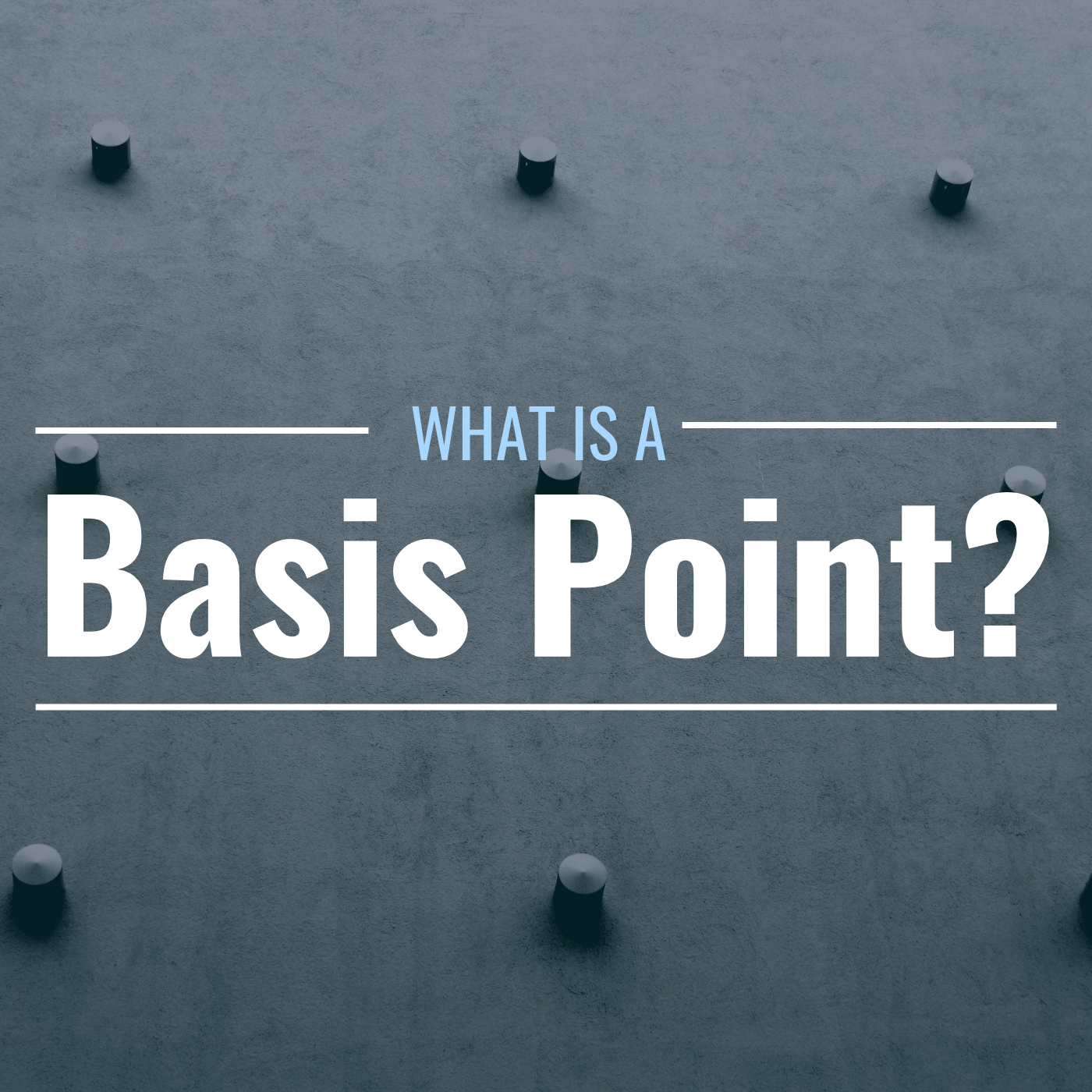# What Is a Basis Point? Definition, Formula & Examples

These tiny measurements make a big impact in the financial worldA basis point is 1/100 of one percent.Andreey from Getty Images; Canva

## What Is a Basis Point?

A basis point is the smallest unit of measurement in finance. Accountants, bankers, and other financial professionals use basis points to discuss amounts that are less than one percent.

One basis point (abbreviated as “bps” and pronounced “beep”) is the equivalent of 1/100 of one percent, or 0.01%. Therefore, a basis point represents a percentage.

### Examples of Basis Points Expressed in Percentage Terms

Basis PointsPercentage Terms

1

0.01%

10

0.10%

50

0.50%

100

1%

1,000

10%

10,000

100%

## Why Are Basis Points Important?

A basis point may be small, but it can have a big impact on the overall economy. For example, if interest rates go up by even a few basis points, that translates to billions, if not trillions, of additional dollars affecting the mortgage industry, credit card rates, and other financial instruments.

Another reason basis points are important is because they are precise. They help clear up some of the confusion that can arise when we discuss the difference between two percentage rates.

For instance, if someone said there has been a 1 percent increase from a 5 percent interest rate, that change could be either a relative change, in numeric value, from 5% to 6%, or it could be an absolute change, from 5% to 5.1%. However, if we instead expressed the increase in basis points, we would know there had been precisely a 100 basis point increase. Thus, the interest rate had increased by 100 basis points to 6%.

## How Are Basis Points Calculated?

To calculate a ratio in basis points, simply multiply the percentage by 100. To calculate a percentage from basis points, simply divide the basis points by 100.

Scroll to Continue

## TheStreet Dictionary Terms

### Basis Point Formulas

Basis Points = Percentage * 100
Percentage = Basis Points / 100

## How Are Basis Points Used?

The Federal Reserve uses basis points to express changes in the fed funds rate. For example, if the Fed raises interest rates by a quarter of a percentage point, it raises them by 0.25 bps.

Interest rate spreads, or the differences between two interest rates, are also denoted in basis points, as are mortgage loans and annuity rate changes.

Expense ratios for mutual funds or ETFs are also expressed in basis points.Other administrative fees and expenses, such as broker commissions, annuity fees, etc. are also expressed in basis points.

## Basis Points and Bonds

However, basis points are used primarily for quantifying changes in bond yields, or the difference in yield between two bonds.

For example, say that a bond's price drops, causing its yield to rise from 6% to 6.10%. One would say its yield rose by 10 basis points.Another example: Say one bond has a yield of 6.5% and another has a yield of 6.75%. The difference can be expressed as 25 basis points.

## Real World Example of Basis Points

In January 2022, yields on the 10-Year Treasury spiked to 1.7% after the consumer price index increased at its fastest pace since 1982. Bond yields move inversely to prices, and this jump was interpreted as an additional sign of inflation being felt through the economy. Yields on the 10-Year Treasury increased by 20 basis points in the first two weeks of January 2022 alone.

## Basis Point Terminology

So, why are they called “basis points” anyway? The term originated with interest rates. Traders would trade the “basis,” which meant the spread between two rates. This was usually a very small percentage, although traders have coined other terms based off basis points to denote larger values, such as:

• MegaBips, or 10 bps (0.1%)
• UltraBips, or 100 bps (1%), and
• GigaBips, or 1000 bps (10%)